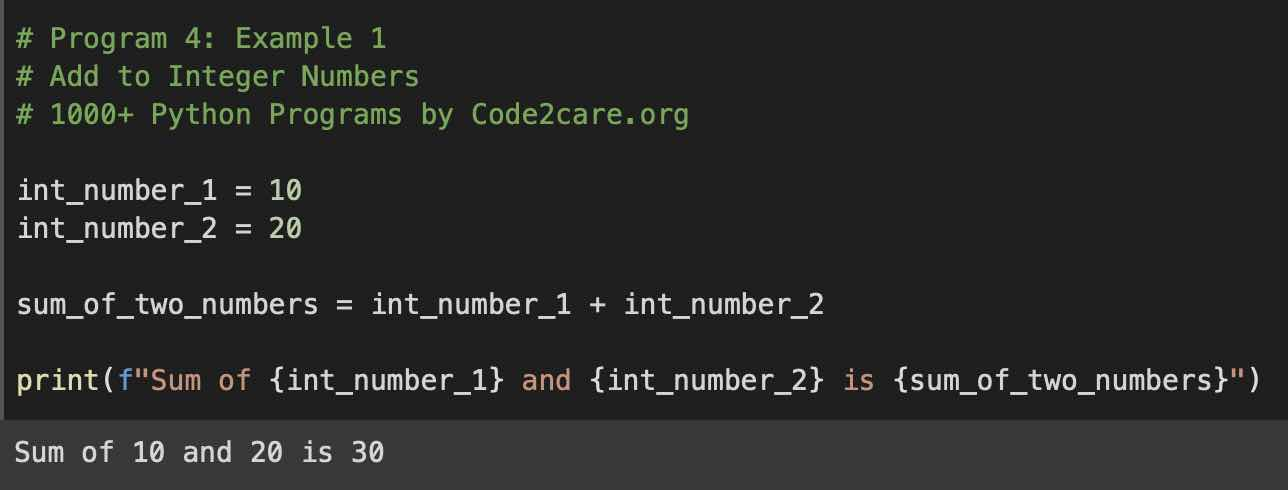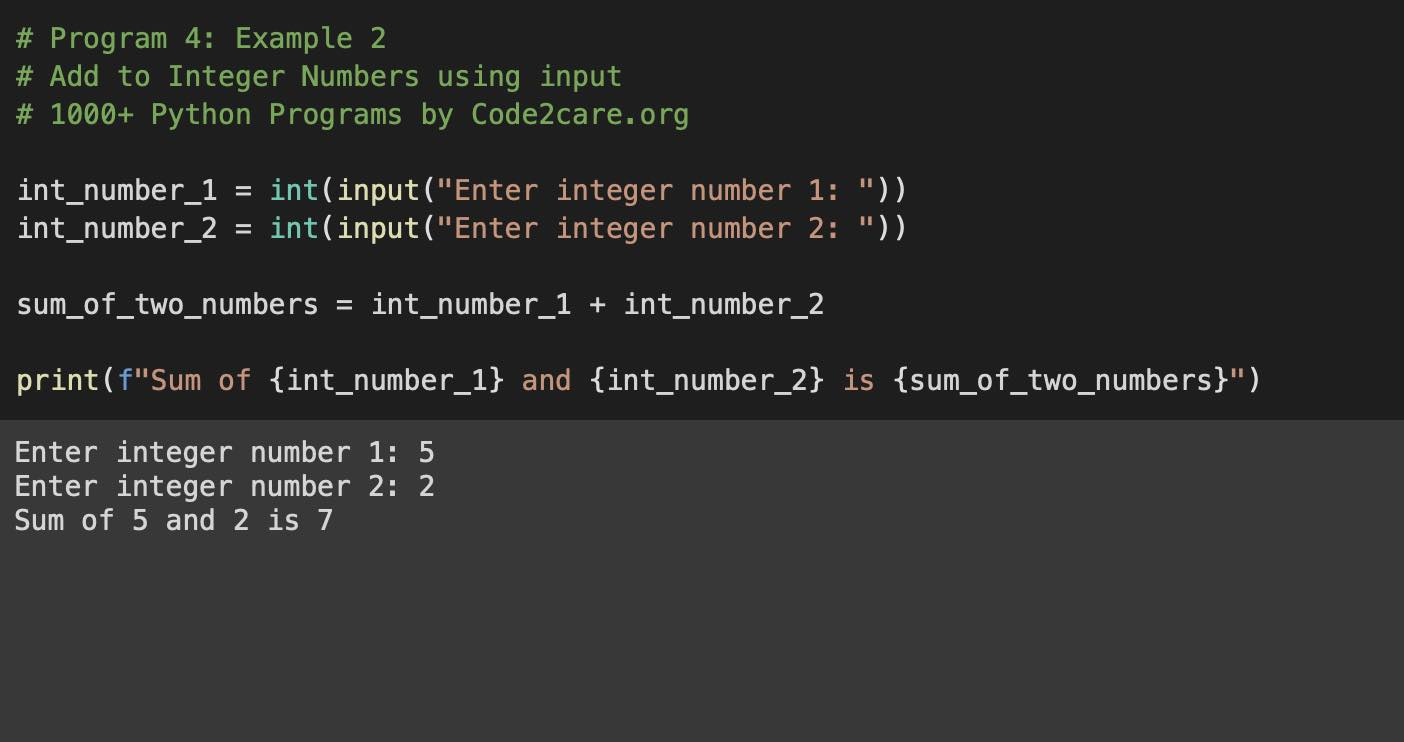# Program 5: Find Sum of Two Integer Numbers - 1000+ Python Programs

Question 5) Write a program in Python to calculate the sum of two integer numbers.

## Code Example 1: Using two hardcoded integer values

``````# Program 5: Example 1
# 1000+ Python Programs by Code2care.org

int_number_1 = 10
int_number_2 = 20

sum_of_two_numbers = int_number_1 + int_number_2

print(f"Sum of {int_number_1} and {int_number_2} is {sum_of_two_numbers}")``````
Output:

Sum of 10 and 20 is 30## Explanation:

1. Create a variable int_number_1 with an integer value hardcoded. Say 10
2. Create a variable int_number_2 with an integer value hardcoded. Say 20
3. Now create a third variable sum_of_two_numbers to hold the sum of two numbers
4. Print the sum using the print function with f-strings formatting.

## Code Example 2: Using user inputs

``````# Program 4: Example 2
# Add to Integer Numbers using input
# 1000+ Python Programs by Code2care.org

int_number_1 = int(input("Enter integer number 1: "))
int_number_2 = int(input("Enter integer number 2: "))

sum_of_two_numbers = int_number_1 + int_number_2

print(f"Sum of {int_number_1} and {int_number_2} is {sum_of_two_numbers}")``````
Output:

Enter integer number 1: 5
Enter integer number 2: 2
Sum of 5 and 2 is 7## Explanation:

We learnt a new in-build function called int() which returns a int value for the provided string.

Functions used:
• input()
• print()
• int()

If an invalid integer value is provided you will get an ValueError error,

``````ValueError Traceback (most recent call last)
<ipython-input-39-77271dff3a60> in <module>
4
5 int_number_1 = int(input("Enter integer number 1: "))
----> 6 int_number_2 = int(input("Enter integer number 2: "))
7
8 sum_of_two_numbers = int_number_1 + int_number_2

ValueError: invalid literal for int() with base 10: '11.1'``````
-

Have Questions? Post them here!